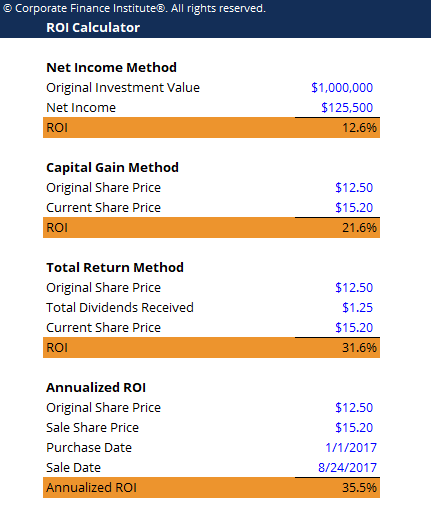Return on Investment Calculator

Return on Investment Calculator

This return on investment calculator will show you how to calculate ROI using four different approaches.

Below is a preview of the return on investment calculator:Enter your name and email in the form below and download the free template now!

Return on Investment Calculator Template

Return on investment (ROI) is a financial ratio used to calculate the benefit an investor will receive in relation to their investment cost, most commonly measured as net income divided by the original cost of the investment. The higher the ratio, the greater the benefit earned.

There are several versions of the ROI formula. The two most commonly used are shown below:

ROI = Net Income / Cost of Investment  or  ROI = Investment Gain / Investment Base

This ROI calculator will demonstrate four ways to obtain the ROI value: net income method, capital gain method, total return method, and annualized ROI.

More Free Templates

For more resources, check out our business templates library to download numerous free Excel modeling, PowerPoint presentation and Word document templates.

• Excel Modeling Templates
• PowerPoint Presentation Templates
• Transaction Document Templates

Financial Analyst Certification

Become a certified Financial Modeling and Valuation Analyst (FMVA)® by completing CFI’s online financial modeling classes and training program!